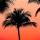Home
IT Knowledge
Inspiration
Languages
EN

# Python - print common elements of two sets

0 points
Created by:Inferio
268

In this article, we would like to show you how to print common elements of two sets in Python.

## Practical example

In this example, we use `intersection_update()` method to keep only the common elements from `my_set1` and `my_set2`.

``````my_set1 = {'A', 'B', 'C'}
my_set2 = {'X', 'B', 'C'}

my_set1.intersection_update(my_set2)

print(my_set1)  # {'B', 'C'}``````

Output:

``{'B', 'C'}``